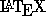Next: Getting Stuff On Top Up: Commands for Math Mode Previous: Special Symbols

## Sequences of Equations

To get a bunch of equations all lined up nicely, you can do it like this with \eqnarray*:

\begin{eqnarray*}
i_w\cdot\psi_v\cdot N_w&=&\psi_w\cdot i_w\cdot N_w\\
&=&\psi_w\cdot i_w\cdot\sum_{\sigma\in G_w}\sigma\\
&=&\psi_w\cdot\sum_{\sigma\in G_w}\sigma\\
&=& i_w\cdot N_w\cdot\psi_w.
\end{eqnarray*}
yieldsA few things to notice are:

• & surrounds the symbol at which the equations are lined up--in this case, the = sign.
• The lines end with \\, which denotes a carriage return.

For more possibilities in formatting your equations, consult theCompanion.

Processed by LaTeX2html